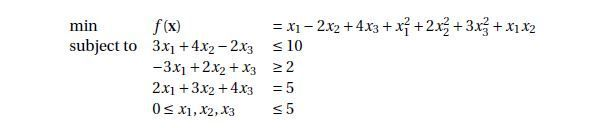## quadratic programming problem with equality and inequality constraints

hi,

I am trying to solve the following problemand I have written the following code:

```proc optmodel;
var x{1..3};
minimize f = x - 2*x + 4*x + x**2 + 2*x**2 + 3*x**2 + x*x;

con r1: 3*x + 4*x -2*x <= 10;
con r2: -3*x + 2*x + x >= 2;
con r3: 2*x + 3*x + 4*x =5;
con r4: x <=5;con r5: x <=5;con r6: x <=5;con r7: x >=0;con r8: x >=0;con r9: x >=0;
solve with qp;
print x;
save qps qpsdata;
quit;```

Please tell me if I am doing it right.Thanks

1 ACCEPTED SOLUTION

Accepted Solutions

## Re: quadratic programming problem with equality and inequality constraints

Your code looks correct to me, but you can do it a little more simply by omitting the SAVE QPS statement and the WITH QP clause and by including the bounds in the VAR statement:

``````proc optmodel;
var x{1..3} >= 0 <= 5;
minimize f = x - 2*x + 4*x + x**2 + 2*x**2 + 3*x**2 + x*x;

con r1: 3*x + 4*x -2*x <= 10;
con r2: -3*x + 2*x + x >= 2;
con r3: 2*x + 3*x + 4*x =5;
solve;
print x;
quit;``````

By using the EXPAND / SOLVE statement, you can see that both versions pass the same formulation to the solver.

## Re: quadratic programming problem with equality and inequality constraints

Your code looks correct to me, but you can do it a little more simply by omitting the SAVE QPS statement and the WITH QP clause and by including the bounds in the VAR statement:

``````proc optmodel;
var x{1..3} >= 0 <= 5;
minimize f = x - 2*x + 4*x + x**2 + 2*x**2 + 3*x**2 + x*x;

con r1: 3*x + 4*x -2*x <= 10;
con r2: -3*x + 2*x + x >= 2;
con r3: 2*x + 3*x + 4*x =5;
solve;
print x;
quit;``````

By using the EXPAND / SOLVE statement, you can see that both versions pass the same formulation to the solver.

Discussion stats